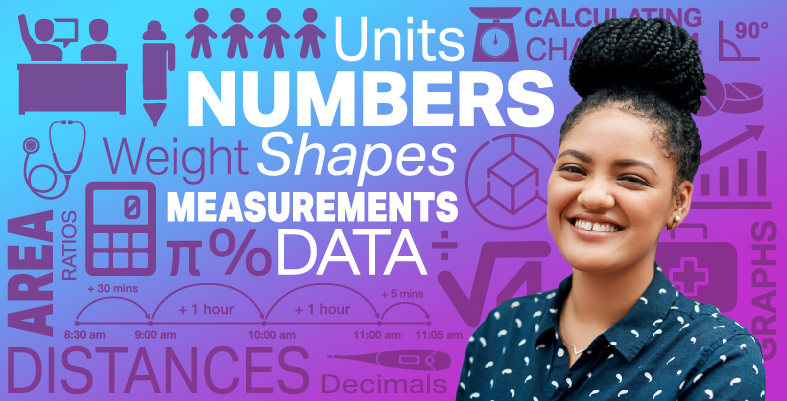Everyday maths for Health and Social Care and Education Support 2

Start this free course now. Just create an account and sign in. Enrol and complete the course for a free statement of participation or digital badge if available.

Free course

# 7 Bringing it all together

Congratulations on completing Everyday maths 2. We hope you have enjoyed the experience and now feel inspired to develop your maths skills further.

Throughout this course you have developed your skills within the following areas:

• understanding and using whole numbers and decimal numbers, and understanding negative numbers in the context of money and temperature

• solving problems requiring the use of the four operations and rounding answers to a given degree of accuracy

• understanding and using equivalences between common fractions, decimals and percentages

• working out simple and more complex fractions and percentages of amounts. Calculating percentage change and using reverse percentages

• adding and subtracting decimals up to two decimal places

• solving ratio problems where the information is presented in a variety of ways

• understanding the order of operations and using this to work with formulas

• solving problems requiring calculations with common measures, including money, time, length, weight, capacity and temperature

• converting units of measure in the same system and those in different systems

• extracting and interpreting information from tables, diagrams, charts and graphs

• collecting and recording discrete data, and organising and representing information in different ways

• finding the mean, median and range of a group of numbers. Finding the mean from grouped data

• using data to assess the likelihood of an outcome and expressing this in different forms

• working with area, perimeter and volume, scale drawings and plans.

FSM_SSH_2